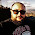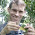## Thursday, November 15, 2012

### Adding a single tip to a tree

A R-sig-phylo member writes:

Dear list members,
I have a large phylogeny to which I need to add branches of a certain length (new taxa) at specific nodes.
I have experimented with bind.tree (ape) and paste.tree (phytools) but they only let you bind trees to together. I can cobble together code that adds a 2 species tree and then erase one of the tips using drop.tip but this is difficult given how the node labels change.
I want to automate this process because I have ~180 additions to make and I want to repeat the additions using branches of different length.
Ideally I would like to have a function where I input the node number to which a branch should be added, the length of that branch, and the name of the tip.
Any suggestions?

It seems like the basic problem is not that bind.tree and paste.tree don't work; but rather that they only attach two bifurcating trees together, and the user wants to attach one tip to a tree.

Well, it turns out that this is not too hard. Here's how, by way of demonstration:

> library(phytools)
> # simulate receptor tree
> tree<-pbtree(n=10)
> plotTree(tree,node.numbers=T)
> # create tip (modify as desired)
> tip<-list(edge=matrix(c(2,1),1,2),
tip.label="species.name",
edge.length=1.0,
Nnode=1)
> class(tip)<-"phylo"
> # attach to any node (say, node 16)
> btree<-bind.tree(tree,tip,where=16)
> plotTree(btree)

Ok, how about writing a function for it? Let's call it bind.tip:

bind.tip<-function(tree,tip.label,edge.length=NULL,where=NULL){
if(is.null(where)) where<-length(tree\$tip)+1
tip<-list(edge=matrix(c(2,1),1,2),
tip.label=tip.label,
edge.length=edge.length,
Nnode=1)
class(tip)<-"phylo"
obj<-bind.tree(tree,tip,where=where)
return(obj)
}

That's it.

1.This comment has been removed by the author.

2.Dear Liam,

I need to add a list of 230 species in a phylogenetic tree. Is there a logical way to add the species to the root of the genus to which it belongs, in a systematic way, that is, to make the function recognizes the name of the genus to which the species belongs, and so it adds the species to that root? I can name the roots of the genre of the tree, if necessary.

1.Hi Thiago. I believe you also posted this question to R-sig-phylo. Was my answer helpful? Liam

2.Hi Liam, thank you. I will try to use your new function on my data.

Thanks,

Thiago.

3.This comment has been removed by the author.

Note: due to the very large amount of spam, all comments are now automatically submitted for moderation.